# How to Prepare Electrostatics for NSEP

###### ByRamraj Saini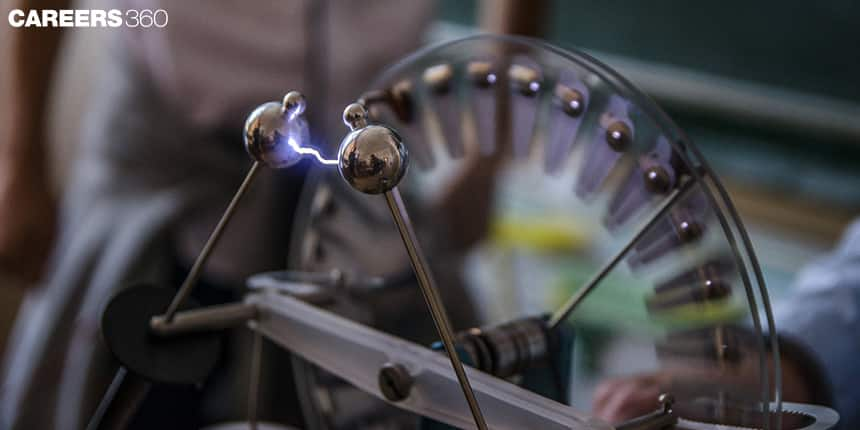###### Synopsis

Electrostatics is an important unit for National Standard Examination in Physics (NSEP). It is a base for other chapters like Electric Current and The Magnetic Effect Of Electric Current. This article contains some important concepts, formulas, and previous year's questions that can help students to prepare and score well in the exam.###### Synopsis

Electrostatics is an important unit for National Standard Examination in Physics (NSEP). It is a base for other chapters like Electric Current and The Magnetic Effect Of Electric Current. This article contains some important concepts, formulas, and previous year's questions that can help students to prepare and score well in the exam.

Matter is made of three elementary particles i.e electrons(e), protons(p), and neutrons(n). Apart from mass, these elementary particles have one more property charge. Electrons are negatively charged particles, protons are positively charged particles and neutrons have zero charges. Usually, matter has an equal number of protons and electrons and therefore it is electrically neutral. But when the number of protons and neutrons are different, matter becomes positively or negatively charged. Electrostatics is part of physics that deals with phenomena and properties of static charge.

## Important Concepts In Electrostatics

Electrostatics is very important because it is the foundation of other chapters. Let's start with the properties of charge.

### Properties of charge

1. Electric charge is a scalar quantity means the sum of charge will be a simple algebraic sum.

2. Charges are quantized particles meaning if electrons and protons are the only charges carried then all observable charges must be an integral multiple of charge on electrons.

3. Charge on a system is always conserved. It means that the net charge on an isolated system remains the same.

4. Similar charges on objects repel each other and unlike charges on the object attract each other.

5. A static charge produces and feels only an electric field.

6. A uniformly moving charge can create and feel an electric field as well as a magnetic field.

7. An accelerated charge emits energy in form of electromagnetic waves.

## Coulomb's Law

Coulomb established a relation that force applied by a charged particle on another charged particle is given by a formula below.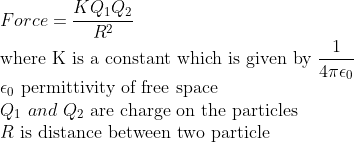## Electric Field and Intensity

A static charge produces an important field around it in space known as an electric field. Due to this electric field, other charges feel the presence of this charge or we can say the other charge experiences a force. The electric field has its own existence and is present if there is no other charge.

The intensity of the electric field is defined as force per unit charge. It means if there is a charge Q and we want to calculate the electric field or intensity of the electric field at a distance R then we calculate just force on the unit charge.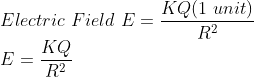This is a formula that is used to calculate the electric field by charge Q at a distance R.

## Electric Potential

It is defined as work done by an external force to carry a unit charge from an infinite distance to a reference point against the electric field. In other words, the potential is potential energy per unit charge. Potential can only be defined with a reference point. This means a charge does not have its own potential. It is defined as relative to others which is a potential difference. We can only calculate the potential differences. Potential is a potential difference between an infinite point and a reference point.

There is a charge Q, we want to calculate the potential at a distance that it is given by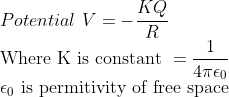## Electric Potential Energy

When work is done by an external force against a conservative force like an electric force, spring force, or gravitation force, the work done by an external force is stored as a potential energy of the system. If the force is electric force then potential energy is known as electric potential energy. Potential energy due to two point charges Q1 and Q2 that are separated by a distance R is given by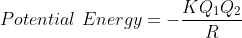There is a relation between the electric field and potential difference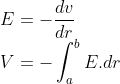## NSEP Previous Year Questions From Electrostatics

Previous year's questions are the best source to know the horizontal spread and vertical depth of any exam. They provide us with a comprehensive understanding of how to prepare for the exam. Following are a few NSEP questions from electrostatics. Students can practice these to command the concepts.

Q-1 (NSEP - 2015-16)

A charge (− 2Q) is distributed uniformly on a spherical balloon of radius R. Another point charge (+ Q) is situated at the center of the balloon. The balloon is now inflated to twice the radius. Neglecting the elastic energy involved in the process, the change in total electric energy of the system is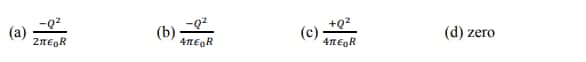Q-2 (NSEP - 2015-16)

A charge +q is placed at a distance of d from a point O. A conducting body surrounds point O such that q remains outside. The electric field at O due to the

Also, Read| How To Prepare Magnetic Effects Of Current

induced charge is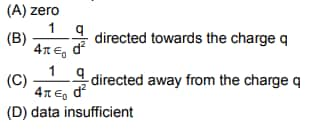Q-3 (NSEP - 2016-17)

The breakdown field for air is about 2 × 106 volt/m. Therefore, the maximum charge that can be placed on a sphere of diameter 10 cm is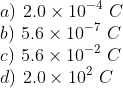Q-4 (NSEP - 2017-18)

Acidified water from certain reservoirs kept at a potential V falls in the form of small droplets each of radius r through a hole into a hollow conducting sphere of radius a. The sphere is insulated and is initially at zero potential. If the drops continue to fall until the sphere is half full, the potential acquired by the sphere is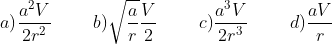## NSEP Study Materials For Electrostatics

• NCERT Class 12 Physics chapter Electrostatics

• NCERT Exemplar problems from Electrostatics

• NSEP previous year papers

• Electrostatics

## Careers360 helping shape your Career for a better tomorrow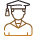#### 250M+

Students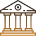#### 30,000+

Colleges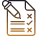#### 500+

Exams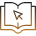E-Books